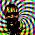## Saturday, July 30, 2011

### Latex for Blogger

A couple of years ago, I posted that I was using htlatex to do math-heavy posts. This worked in a pinch, the equations are all nicely rendered as *.png’s and they have all the normal Latex auto-numbering and cross-referencing. This only works for posts though, no equation support in comments. I learned about MathJax, which is a much better option than non-scaling pngs for math, and it supports equations in comments. All it requires is adding a script to the blogger template file.

<script src=’http://cdn.mathjax.org/mathjax/latest/MathJax.js?config=TeX-AMS-MML_HTMLorMML’ type=’text/javascript’>
</script>

Then run htlatex with the mathml option.

[jstults@grafton]\$ htlatex foo.tex ”xhtml,mathml”

This turns foo.tex into foo.html, which has the equations in MathML markup. MathJax renders the MathML so everything looks pretty.

MathJax also renders any Latex it finds on the page (including in comments!). The normal single dollar sign for inline math does not work, since this would cause trouble if people were trying to discuss money. Here's what does work though:

% inline math in comments use:
$$x + y = z$$
$x + y = z \ % or (for numbered equations): \begin{equation} x + y = z \end{equation} inline in comments: \ ( x + y = z \) A couple numbered equations in the post \begin{equation} \frac{\partial x}{\partial t} = A x \end{equation} foo \begin{equation}\frac{\partial y}{\partial t} = g(y) + f \end{equation} #### 4 comments: 1.test inline $$1/\sqrt{n}$$ display \[ x + y = x$
numbered equation
\begin{equation} x + y = z \end{equation}

2.well \ ( ) \ for inline seems to work (remove space between the opening \ and ) and the closing \ and )

numbering doesn't seem to work in comments though

3.Does this parse?

$$\Phi = \varphi - \int_{t^{_{0}}}^{t}f\left ( \tau d\tau \right )$$

4.KPZ equation

$\partial_t h = \nu \nabla^2 h + \lambda (\nabla h)^2 + \eta(x,t)$